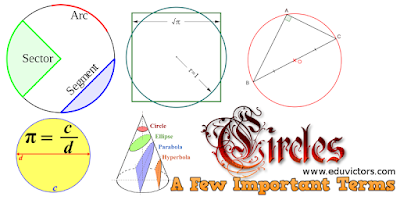## Chapter 10 - Circles - Important Terms You Should Know

1. A circle is a collection (set) of all those points in a plane, each one of which is at a constant distance from a fixed point in the plane.

2. The fixed point is called the centre and the constant distance is called the radius of the circle.

3. All the points lying inside a circle are called its interior points and all those points which lie outside the circle are called its exterior points.

4. The collection (set) of all interior points of a circle is called the interior of the circle while the collection of all exterior points of a circle is called the exterior of the circle.

5. A line segment joining two points on a circle is called the chord of the circle.

6. A chord passing through the center of the circle is called a diameter of the circle.

7. A line which meets a circle in two points is called a secant of arcthe circle.

8. A polygon is a closed figure made up of three or more line segments (sides) such that each line segment intersects exactly two others at its end – points (vertices) and no two line segments which intersect are collinear.

9. A polygon is called a regular polygon, if it has all its sides equal and has all its angles equal.

10. A (continuous) part of a circle is called an arc of the circle. The arc of a circle is denoted by the symbol ‘’.

11. Circumference: The whole arc of a circle is called the circumference of the circle.

12. Semi-circle: One – half of the whole arc of a circle is called a semi – circle of the circle.

13. Minor and Major arcs: An arc less than one - half of the whole arc of a circle is called a minor arc of the circle, and an arc greater than one – half of the whole arc of a circle is called a major arc of the circle.

14. Central Angle: Any angle whose vertex is centre of the circle is called a central angle.

15. Degree measure of an Arc: The degree measure of a minor arc is the measure of the central angle subtended by the arc.

16. Congruent Circles: Two circles are said to be congruent if and only if either of them can be superposed on the other so as to cover it exactly.

17. Congruent Arcs: Two arcs of a circle (or of congruent) circles) are congruent if either of them can be superposed on the other so as to cover it exactly.

18. Sector of a circle: The part of the plane region enclosed by an arc of a circle and its two bounding radii is called a sector of a circle.

19. Segment of a circle: A chord of a circle divides it into two parts. Each part is called a segment.

20. The part containing the minor arc is called the minor segment, and the part containing the major arc is called the major segment.

21. A quadrilateral, all the four vertices of which lie on a circle is called a cyclic quadrilateral. The four vertices A, B, C and D are said to be Concyclic points.